# Reasoning Quiz For RRB NTPC : 16th January 2020 of figure and statement-conclusion

Q1. 18, 19, 22, 27, ?
(a) 33
(b) 34
(c) 36
(d) 32

Q2. In the following figure, the triangle represents teachers, the circle represents students and the rectangle represents actors. Which number represents teachers who are also students and actors ?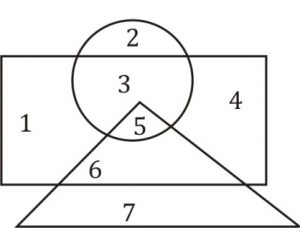(a) 3
(b) 6
(c) 5
(d) 2

Q3. Find the number of triangles in the given figure: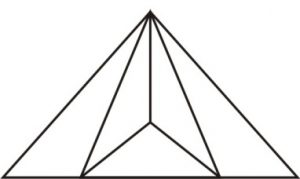(a) 6
(b) 7
(c) 8
(d) 9

Q4. Which answer figure will complete the pattern in the question figure: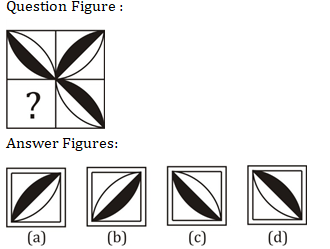Q5. Select the answer figure in which the questions figure are hidden/embedded.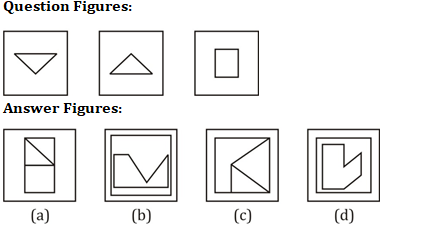Q6. Which of the answer figures is exactly the mirror image of the given figure, when the mirror is held on the line MN ?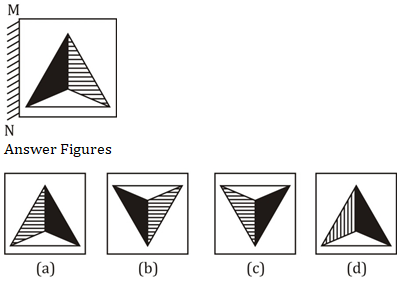Q7. What figure will come next.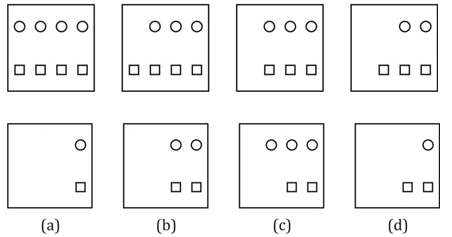Q8. In each of the following question below are given some statements followed by some conclusions. Taking the given statements to be true even if they seem to be at variance from commonly known facts, read all the conclusions and then decide which of the given conclusion logically follows the given statements.
Statements: I. Some pins are cups.
II. No cup is book.
Conclusions: I. Some pins are books.
II. Some pins are not books.
(a) Only conclusion (I) follows
(b) Only conclusion (II) follows
(c) Both conclusion follow
(d) Neither conclusion (I) nor conclusion (II) follows

Q9. Statement is given followed by three conclusions I, II and III, You have to consider the statement to be true even they seem to be at variance from commonly known facts. You have to decide which of the given conclusions, if any, follows from the given statement.
Statement: Comic books contain pictures.
Conclusions:
(I) All books contain pictures.
(II) Books may or may not contain pictures.
(III) Books other than the comic books do not contain pictures.
(a) Only conclusion I follows.
(b) Only conclusion II follows.
(c) Both conclusions I and II follow.
(d) Neither Conclusion follows.

Q10. Directions: Two statements are given followed by two conclusions/ Assumptions, I and II. You have to consider the statements to be true, even if it seems to be at variance from commonly known facts. You are to decide which of the given conclusions/ assumptions can definitely be drawn from the given statement.
Statements
1. All children are students.
2. All students are players.
Conclusions
I. All cricketers are students.
II. All children are players.
(a) Only conclusion I follows.
(b) Neither conclusion I nor II follows.
(c) Both conclusions I and II follow.
(d) Only conclusion II follows.

#### Solutions:

S1. Ans.(b)
Sol.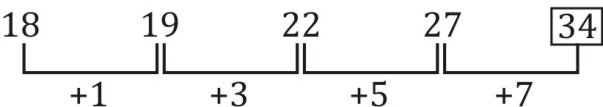Difference is odd number.

S2. Ans.(c)
Sol.
The required region should be common in all the three figures. Such region is represented by ‘5’.

S3. Ans.(b)
Sol.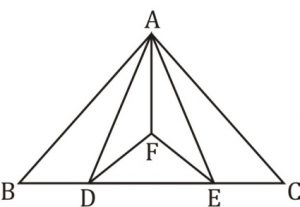S4. Ans.(b)
Sol.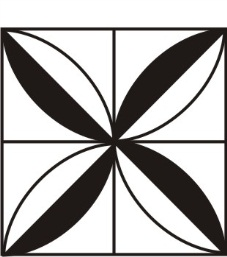S5. Ans.(a)
Sol.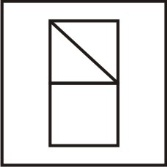S6. Ans.(a)
Sol.

S7. Ans.(b)
Sol.

S8. Ans.(b)
Sol.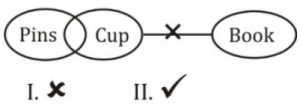Only conclusion II follows.
S9. Ans.(b)
Sol.
Only conclusion II follows. Comic books and some other books may contain pictures. Some books do not contain pictures.

S10. Ans.(d)
Sol.
Both Premises are Universal Affirmative (A-type).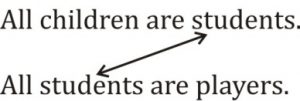A + A ⇒ A – type of Conclusion
“All children are players”.
This is Conclusion II.

###### RRB NPTC 2019-20 | Reasoning | Missing Number in The Figure Tricks

Important Links for RRB NTPC Recruitment 2019